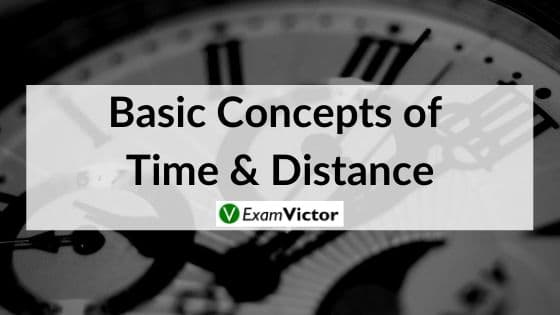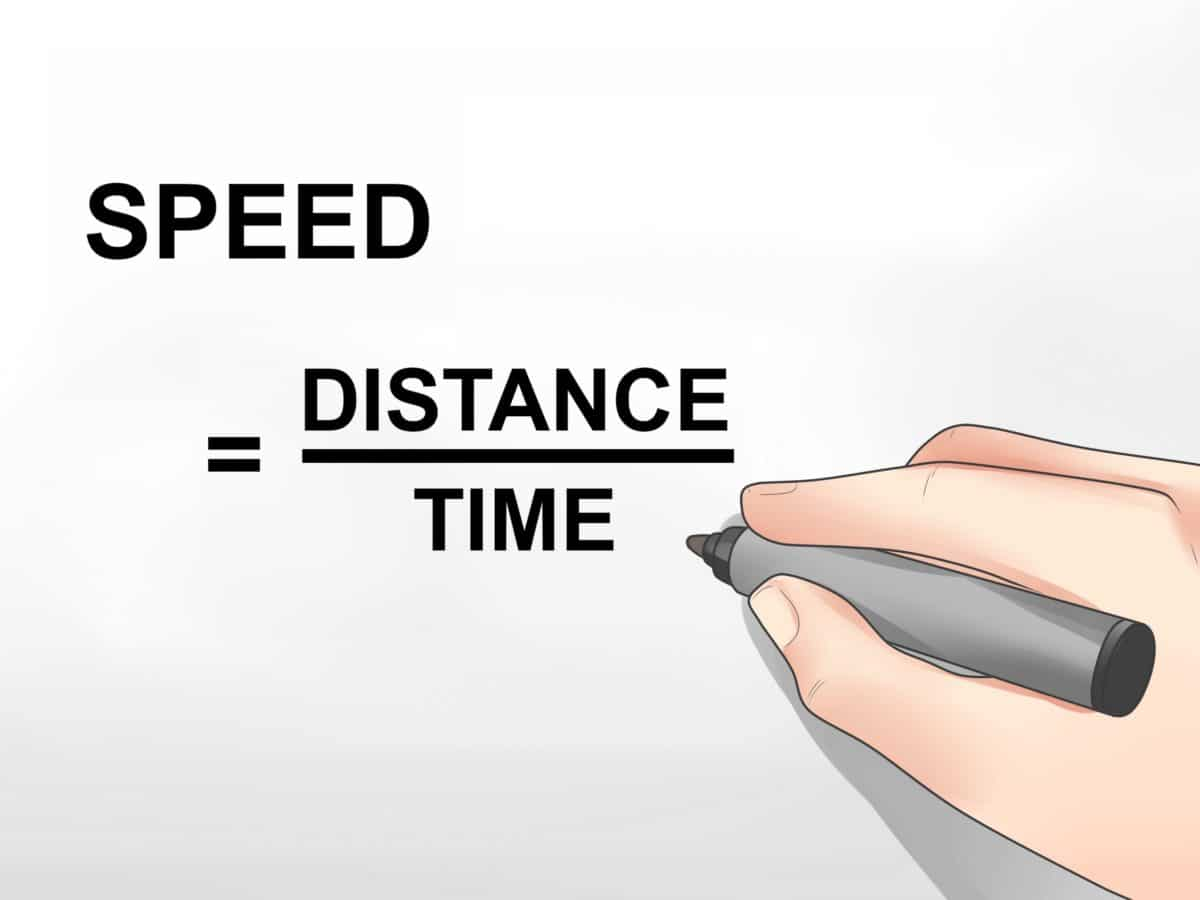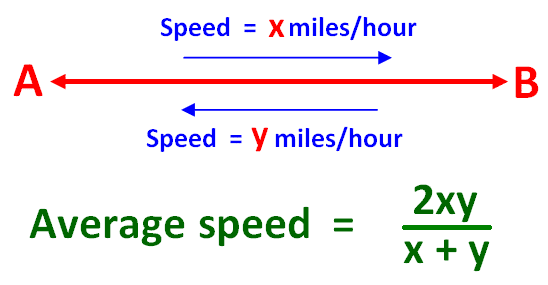# Basic Concepts of Time and DistanceLast Updated on Nov 3, 2020

Speed is a very basic concept in motion which is all about how fast or slow any object moves. We define speed as distance divided by time. In this article, we will discuss the speed, time and distance aptitude questions. As this topic forms an integral part of every competitive exam, you cannot afford to miss this topic. This article will equip you to solve all time speed and distance word problems.

There is a relationship between speed, distance and time:• Using this formula, all basic problems can be handled. However, you need to make sure about the correct usage of units while using the above formulas.
• Speed is inversely proportional to the time taken when the distance travelled is constant. So when speed increases, time decreases and vice versa.

Let us take a look at some simple examples of distance, time and speed problems.

Example 1. A boy walks at a speed of 4 kmph. How much time does he take to walk a distance of 20 km?

Solution

Time = Distance / speed = 20/4 = 5 hours.

Example 2. If a person walks at 4 mph, he covers a certain distance. If he walks at 9 mph, he covers 7.5 miles more. How much distance did he actually cover?

Now we can see that the direct application of our usual formula Distance = Speed * Time or its variations cannot be done in this case and we need to put in extra effort to calculate the given parameters.

Let us see how this question can be solved.

Solution

For these kinds of questions, a table like this might make it easier to solve.

 Distance Speed Time d 4 t d+7.5 9 t

Let the distance covered by that person be ‘d’.
Walking at 4 mph and covering a distance ‘d’ is done in a time of ‘d/4’
If he walks at 9 mph, he covers 7.5 miles more than the actual distance d, which is ‘d+7.5’.
He does this in a time of (d+7.5)/9.

Since the time is same in both the cases →
d/4 = (d+7.5)/9            →        9d = 4(d+7.5)   →        9d=4d+30        →        d = 6.

So, he covered a distance of 6 miles in 1.5 hours.

### Average Speed

Suppose you and your friend decided to take your brand new red sports car out for a spin. Your car is capable of speeds up to 220 mph. You drove 45 miles in 1.25 hours. At the end of your trip, your friend tells you that your average speed during the trip is 36 miles per hour. You were appalled. You asked yourself, ‘How can a sports car have such a pitiful average speed?’ and tried to recall how much you paid for it. What is average speed anyway?

The average speed of an object is the total distance travelled by the object divided by the elapsed time to cover that distance. It’s a scalar quantity which means it is defined only by magnitude. A related concept, average velocity, is a vector quantity. A vector quantity is defined by magnitude and direction. For example, we might say that a car has an average speed of 25 miles per hour. Its average velocity might be 25 miles per hour due east.

Average speed can be viewed as the rate of change in distance with respect to time. A car travelling at an average speed of 25 miles per hour covers an average distance of 25 miles every hour.###### Case 1: When the distance is constantWhere x and y are the two speeds at which the same distance has been covered. Applicable when one travels at speed a for half the distance and speed b for other half of the distance. In this case, the average speed is the harmonic mean of the two speeds. On similar lines, you can modify this formula for one-third distance.

Example: Sheena drove at an average speed of 30 miles per hour for T hours and then at an average speed of 60 miles/hr for the next T hours. If she made no stops during the trip and reached her destination in 2T hours, what was her average speed in miles per hour for the entire trip?

(A)   40
(B)   45
(C)   48
(D)   50
(E)    55

Solution: Here, the time for which Sheena travelled at the two speeds is the same.
Average Speed = (a + b)/2 = (30 + 60)/2 = 45 miles per hour

##### Average speed = (x + y)/2
Where x and y are the two speeds at which we travelled for the same time. Applicable when one travels at speed a for half the time and speed b for another half of the time. In this case, the average speed is the arithmetic mean of the two speeds.

Example: Sheena drove at an average speed of 30 miles per hour for the first 30 miles of a trip & then at an average speed of 60 miles/hr for the remaining 30 miles of the trip. If she made no stops during the trip what was her average speed in miles/hr for the entire trip?

(A) 35
(B) 40
(C) 45
(D) 50
(E) 55

Solution: Here, the distance for which Sheena travelled at the two speeds is the same.

Average Speed = 2xy/(x+y) = 2*30*60/(30 + 60) = 40 mph

### Summary

In this blog post, we have provided the basic concepts of Time and Distance with the help of easy examples and detailed explanation. For post related to Linear Equation, click the given link.
Hope you liked this post.  Let us know your views in the comment section below.